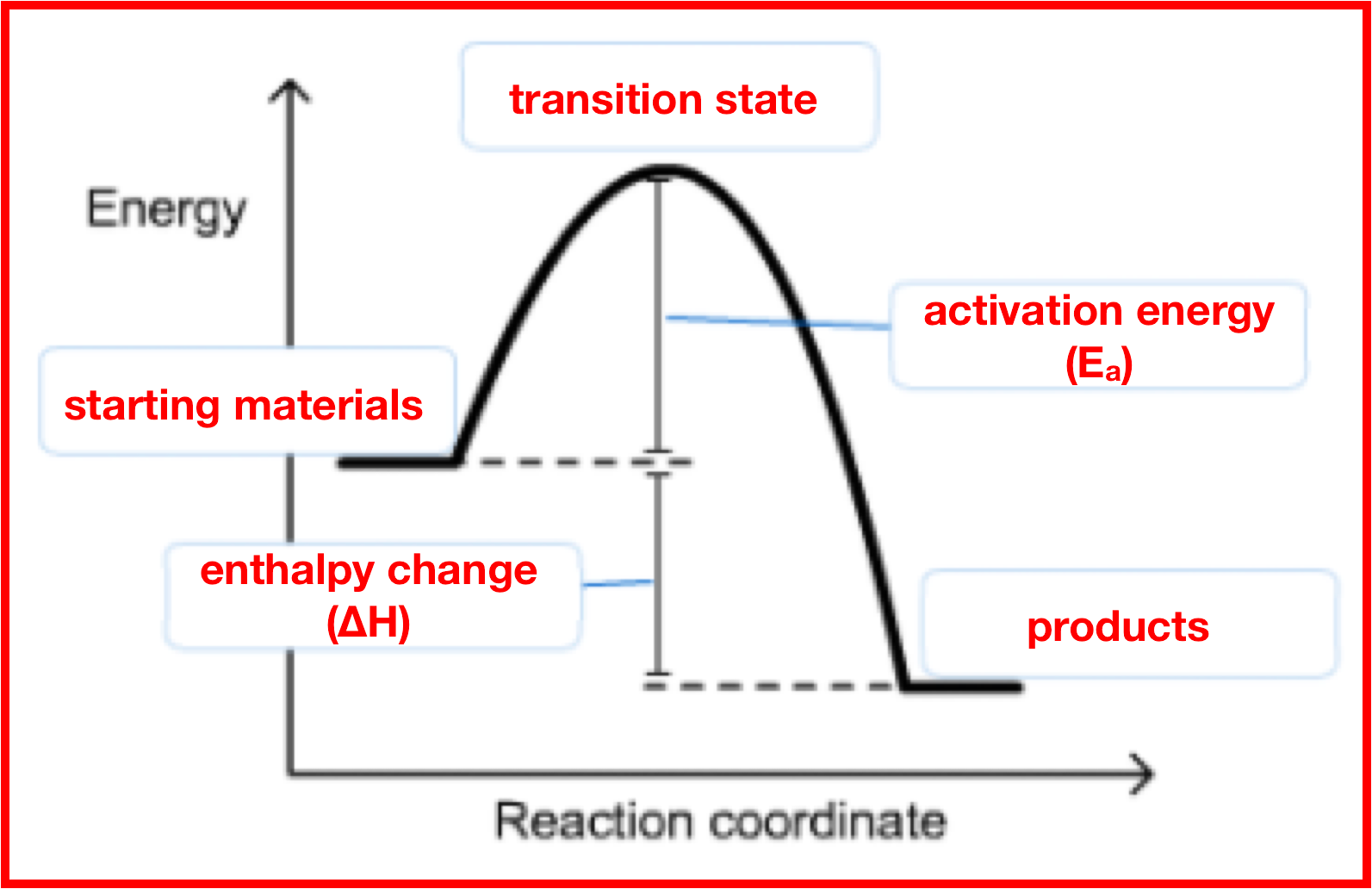# Problem: The activation energy for some reaction X2 (g) + Y2 (g) → 2XY (g)is 167 kJ/mol, and ΔE for the reaction is 128 kJ/mol. What is the activation energy for the decomposition of XY?

###### FREE Expert Solution

Recall that for energy diagrams:If we need to calculate for the EA of the decomposition reaction (reverse reaction), we just have to take the difference of ΔE or ΔH and the EA of the forward reaction

89% (424 ratings)###### Problem Details

The activation energy for some reaction

X2 (g) + Y2 (g) → 2XY (g)

is 167 kJ/mol, and ΔE for the reaction is 128 kJ/mol. What is the activation energy for the decomposition of XY?

Frequently Asked Questions

What scientific concept do you need to know in order to solve this problem?

Our tutors have indicated that to solve this problem you will need to apply the Energy Diagram concept. If you need more Energy Diagram practice, you can also practice Energy Diagram practice problems.

What professor is this problem relevant for?

Based on our data, we think this problem is relevant for Professor Perrin's class at UCSD.

What textbook is this problem found in?

Our data indicates that this problem or a close variation was asked in Chemistry: An Atoms First Approach - Zumdahl Atoms 1st 2nd Edition. You can also practice Chemistry: An Atoms First Approach - Zumdahl Atoms 1st 2nd Edition practice problems.